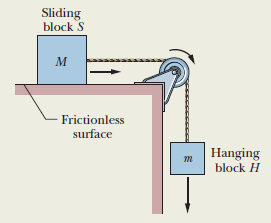# Problem: Suppose that in the figure, the masses of the blocks are 2.0 kg and 4.0 kg.(a) Which mass should the hanging block have if the magnitude of the acceleration is to be as large as possible?(b) What then are the magnitude of the acceleration and(c) the tension in the cord?

###### FREE Expert Solution
84% (303 ratings)
###### Problem Details

Suppose that in the figure, the masses of the blocks are 2.0 kg and 4.0 kg.
(a) Which mass should the hanging block have if the magnitude of the acceleration is to be as large as possible?
(b) What then are the magnitude of the acceleration and
(c) the tension in the cord?